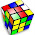## Friday, May 09, 2008

### Mothers' Day Puzzle for all our Supermoms

Take the following mathematical equation:

MOM2 = AMAZON

Can you replace each letter with a different digit {0 to 9} so that the equation makes sense? The letter will represent that digit everywhere the letter appears.

1.Should I assume that the LH side of the equation is (MOM)squared, or M*0*Msquared??

2.3.Come on... it's not that hard. And to clarify once again, that is the letter 'O' which can be any digit, not just zero.

4.Well, I have the answer. But is there a less tedious solution than working through the multiplication digit by digit?

5.Obviously if M and O are set, then the other values are decided. The smallest six-digit square with different digits is 323^2. The largest is 989^2. There are also things you can notice like M can't be 5 or 6, so that narrows things down.